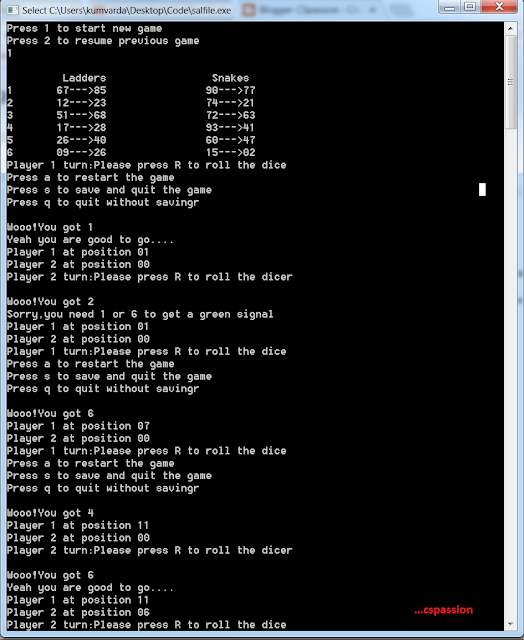## Snakes and Ladder game implementation in C(without GUI)

On 01:50 by Vardan Kumar in    No comments

## FUNCTIONALITIES IN THE GAME

• Use of File handling to save the game and resuming it from previously save point.
• Random selection of six different combinations of snakes as well as six different ladders every time the game runs.
• A player gets a green signal only if he gets 1 or 6 as dice value.
•  A player gets a consecutive turn if he gets 6 as dice value

•Snakes and Ladders in C
• Player is promoted to an end point of the ladder he hits(off course if hits at a particular position).
• Player is demoted to an end point of the snake he hits(off course if hits at a particular position).Player hit by snake and ladder
• A player can only win if he reaches 100 not more or less,for example if he is at 99 and he gets 5,then he is not allowed to move his piece,he can only win if he gets 1).
• A lot more logical constraints are applied so that the logical feasibility of the game is maintained.

### SOURCE CODE

#include<stdio.h>
#include<stdlib.h>
#include<time.h>
void dissal(int pl,int comp)
{
if(pl<comp)
{
printf("Yipee you got a ladder to %d",comp);
}
else if(pl>comp)
{
printf("oops snake has eaten you to position %d",comp);
}
}
int checkforsal(int player)
{
int i,pos;
for(i=0;i<6;i++)
{
{
break;
}
else if(player==snaup[i])
{
pos=snalow[i];
break;
}
else
{
pos=player;
}
}
return pos;
}
void main()
{
int temp,p1=0,p2=0,di=0,fp1=0,fp2=0,fturn1=1,pocket=0,i;
int j=0;
FILE *f;
char ch,get;
int state=0;
while(1)
{
printf("Press 1 to start new game\n");
printf("Press 2 to resume previous game\n");
fflush(stdin);
srand(time(NULL));
get=getchar();
if(get=='1')
{
state=0;
}
else if(get=='2')
{
state=1;
}
else
{
continue;
}
if(state==1)
{
f=fopen("sal.txt","r");
fclose(f);
}
else if (state==0)
{

for( i = 0 ; i < 6 ; i++ )

{

j=0;

snalow[i]=(rand()%90)+1;
snaup[i]=((snalow[i]+(rand()%100)+1)%99)+1;
{
}
if(snalow[i]>snaup[i])
{
temp=snalow[i];
snalow[i]=snaup[i];
snaup[i]=temp;
}
{
}
if(snaup[i]-snalow[i]<9&&snaup[i]<90)
{
snaup[i]=snaup[i]+7;
}

{
i=i-1;
continue;
}
while(j<i)
{
{
i=i-1;
continue;

}
j++;
}
}
}
for(i=0;i<6;i++)
{
printf("\t\t%02d--->%02d\n",snaup[i],snalow[i]);
}
while(p1!=100&&p2!=100)
{
if(fturn1==1)
{
printf("Player 1 turn:Please press R to roll the dice");
printf("\nPress a to restart the game ");
printf("\nPress s to save and quit the game");
printf("\nPress q to quit without saving");
fflush(stdin);
ch=getchar();
if(ch=='r')
{
di=(rand()%6)+1;
printf("\nWooo!You got %d ",di);
if(p1==0&&(di==1||di==6))
{
fp1=1;
printf("\nYeah you are good to go....");
}
if(fp1==1&&di==6)
{
fturn1=1;
p1=p1+6;
pocket=checkforsal(p1);
dissal(p1,pocket);
p1=pocket;
}
else if(fp1==1&&di!=6)
{
fturn1=0;
p1=p1+di;
pocket=checkforsal(p1);
dissal(p1,pocket);
p1=pocket;
}
else if(fp1==0)
{
printf("\nSorry,you need 1 or 6 to get a green signal");
fturn1=0;
p1=0;
}
if(p1>100)
{
printf("Sorry you cannot cross 100");
p1=p1-di;
}
printf("\nPlayer 1 at position %02d\n",p1);
printf("Player 2 at position %02d\n",p2);
}

else if(ch=='q')
{
exit(0);
}
else if(ch=='a')
{
state=0;
p1=0;
p2=0;
fp1=0;
fp2=0;
fturn1=1;
break;
}

else if(ch=='s')
{
state=1;
f = fopen("sal.txt", "w");
//printf("values are %d %d",snalow,snalow);
if(f==NULL)
printf("Unable to open file\n");
fwrite(snaup, sizeof(snaup),1 , f);
fwrite(snalow, sizeof(snalow), 1 , f);
fwrite(&p1,sizeof(int),1,f);
fwrite(&p2,sizeof(int),1,f);
fwrite(&fp1,sizeof(int),1,f);
fwrite(&fp2,sizeof(int),1,f);
fclose(f);
exit(0);
}
else
{
continue;
}
}
else
{
printf("Player 2 turn:Please press R to roll the dice");

fflush(stdin);
if(getchar()=='r')
{
di=(rand()%6)+1;
printf("\nWooo!You got %d ",di);
if(p2==0&&(di==1||di==6))
{
fp2=1;
printf("\nYeah you are good to go....");
}
if(fp2==1&&di==6)
{
fturn1=0;
p2=p2+6;
pocket=checkforsal(p2);
dissal(p2,pocket);
p2=pocket;
}
else if(fp2==1&&di!=6)
{
fturn1=1;
p2=p2+di;
pocket=checkforsal(p2);
dissal(p2,pocket);
p2=pocket;
}
else if(fp2==0)
{
printf("\nSorry,you need 1 or 6 to get a green signal");

fturn1=1;
p2=0;
}
if(p2>100)
{
printf("Sorry you cannot cross 100");
p2=p2-di;
}
printf("\nPlayer 1 at position %02d\n",p1);
printf("Player 2 at position %02d\n",p2);

}
else

{
continue;
}
}
}

if(p1==100)
{
printf("\t\t\tPLayer 1 Wins");
}
else if(p2==100)
{
printf("\t\t\tPlayer 2 Wins");
}
else
{
printf("\nThe game has been restarted\n");
}
}
}